Related Articles

# GRE Geometry | Three – Dimensional Figures

• Last Updated : 25 Apr, 2019

An object which has only height and length are called 2-dimensional figures while an abject which has height length and width are called 3-dimensional object.

Examples of 2-D figures:

Examples of 3-dimensional figures:

1. Cube: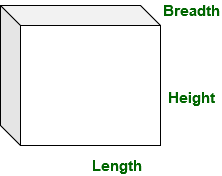In a cube length breadth and height are all equal.
`length = breadth = height `

let’s length, breadth and height as a.
It has 6 square face each with side length a.

```Volume of cube = a * a * a
Volume of cube = a3

Surface area of cube = 6 * area of one face
Surface area of cube = 6 * a2 ```
2. Cuboid: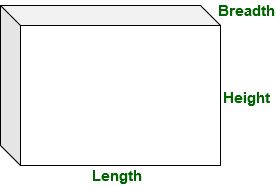In a cuboid length breadth and height are not equal.
It has 6 square face,
```Volume of cuboid = length * breadth * height

Surface area of cuboid = 6 * area of one face
Surface area of cuboid = length * breadth
+ length * height
+ length * height

Surface area of cuboid = 2(length * breadth
+ length * height ) ```
3. Sphere: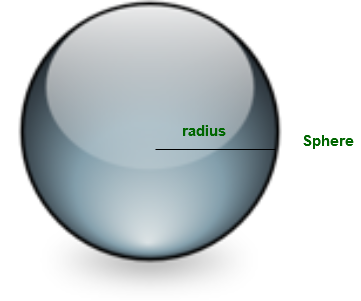A sphere has radius, volume and surface area:
```Volume of sphere = (4 / 3) * π * radius * radius * radius
Volume of sphere = (4 / 3) * π * r3

Surface area of sphere = 4 * &pi * radius * radius
Surface area of sphere = 4 * &pi * radius2

Surface area of sphere = 2 * &pi * radius * radius ```
4. Cylinder: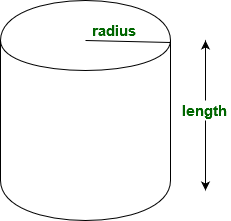A cylinder is a solid or hollow circular shape object with two circular base.
```Volume of cylinder = π * radius * radius * h
Curved surface area of cylinder = 2 * π * radius * h
Total surface area = 2 * π * radius * h + 2 * &pi * radius * radius ```
5. Cone: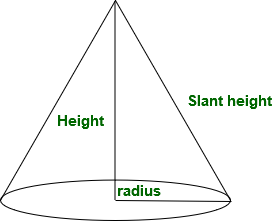A cylinder is a solid or hollow with one circular base and which tappers from base to a point.
```Volume of a cone  = (1 / 3)π * radius * radius * height

Curved surface area of a cone
= π * radius * slant height

Total surface area of cone
= π * radius * slant height
6. Pyramid: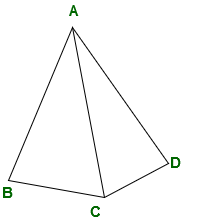Pyramid is 3-d object with polygon base, this polygon base is connected to an apex in pyramid.
```Volume of a pyramid
= (area of base * height) / 3 ```

Examples:

1. What will be the curved surface area of a cylinder having radius 5cm and height 10cm?
```Curved surface area of a cylinder
= 2 * π * r * height
= 2 * 22 / 7 * 5 * 10
= 314.285714286 cm2 ```
2. What will be the slant height of a cone which have curved surface area 990 cm2 and radius 5 cm?
```Curved surface area of a cone = π * radius * slant height
π * radius * slant height  = (22 / 7) * 5 * l
l = 63cm ```
3. What is the volume of a square base pyramid having base side 4 cm and height 6cm?
```Volume of a square
= area of base * height / 3
= 42 * 6 / 3
= 16 * 6 / 3
= 32 cm3 ```
4. What will be the volume of a sphere having radius 2.1cm
```Volume of a sphere
= 4 * π * radius3 / 3
= (4 / 3) * (22 / 7) * 2.13
= 38.808 cm3  ```
5. What will be the surface area of a cube having side lenghth breadth and height 8 cm?
```Surface area of a cube
= 6 * side2
= 6 * 82
= 6 * 64
= 384 cm2 ```
My Personal Notes arrow_drop_up